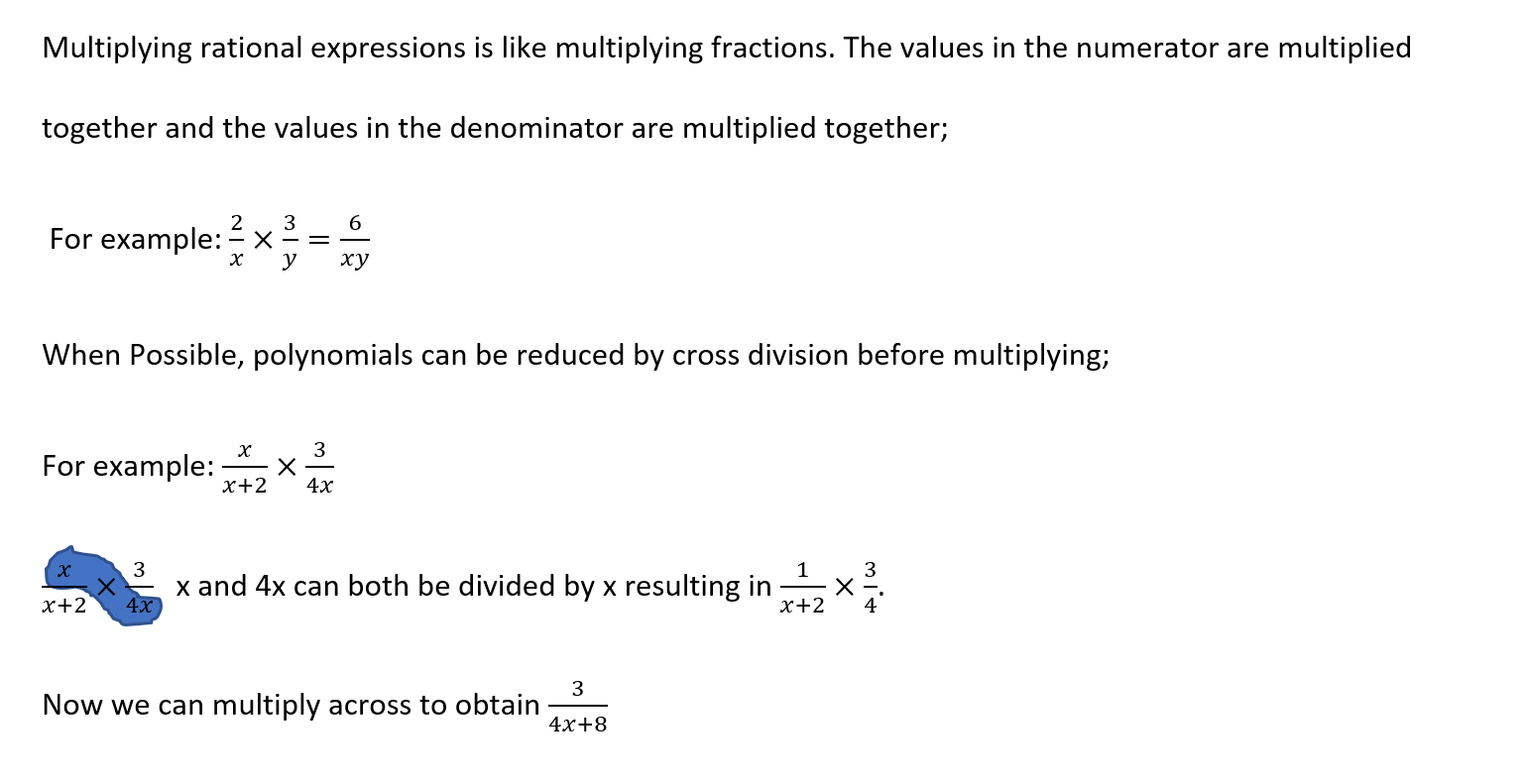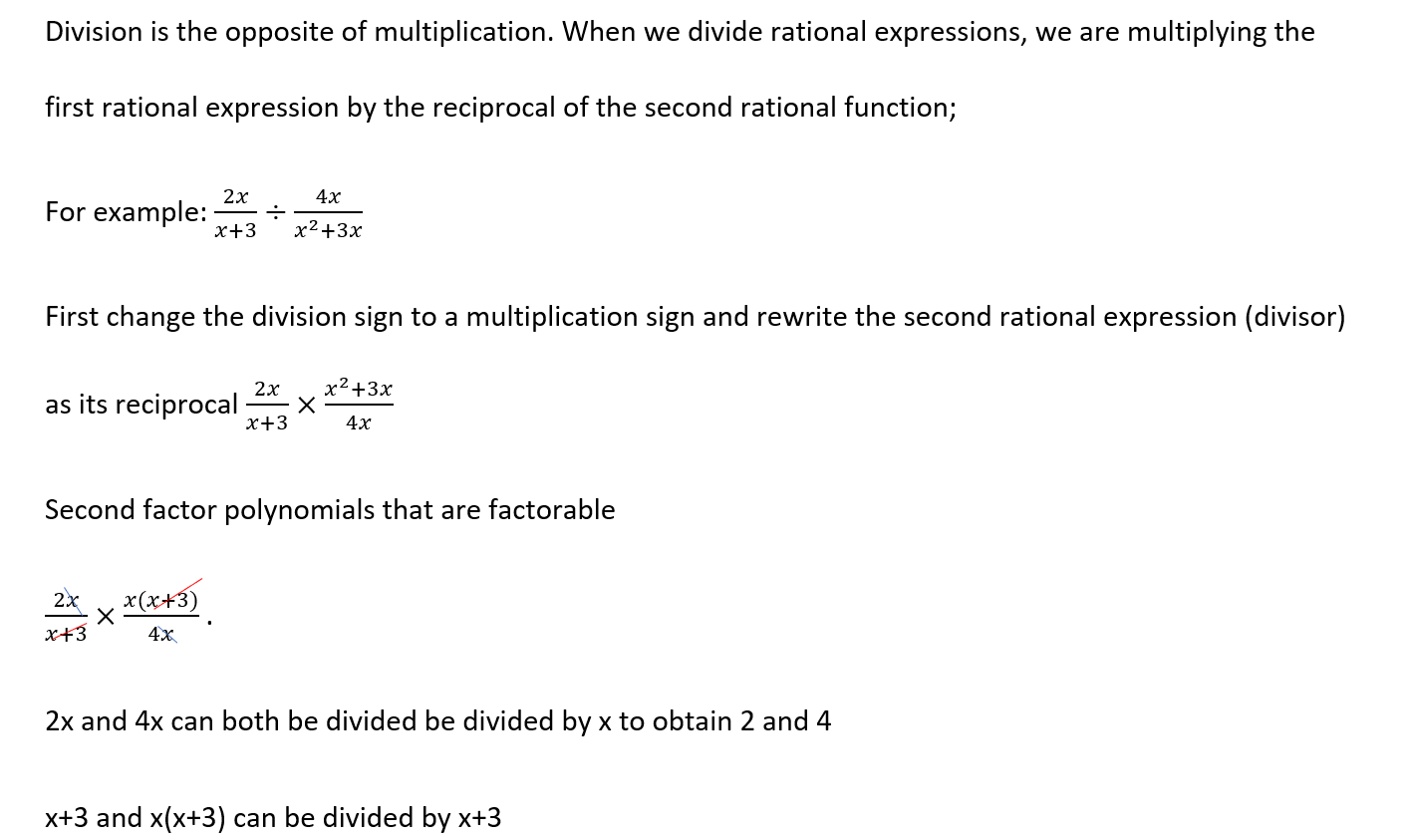Review

What is a Rational Equation?

A rational equation is an equation containing at least one fraction whose numerator and denominator are polynomials

What is a Rational Function?

A rational function is any function which can be defined by a rational fraction, i.e. an algebraic fraction such that both the numerator and the denominator are polynomials.

What makes a Rational Function undefined?

A rational function is undefined when the denominator is equal to zero. On SAT you may be asked to find the value of a variable that makes a rational expression undefined.

How to find the x and y intercepts of an Rational function.

To find the x-intercept, make the numberator of the rational function equal to zero. To find the y intercept, substitute zero for the value of x in the equation.

What is a Rational Inequality?

A rational Inequality is an Inequality containing at least one fraction whose numerator and denominator are polynomialsMultiplying and dividing rational expressions